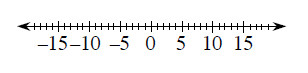### Home > MC2 > Chapter 4 > Lesson 4.3.4 > Problem4-112

4-112.

Copy the following problems, then use the number line to help you fill in $<$ (less than) or $>$ (greater than) on the blank line.Refer to problem 4-89 for help on all parts.

1. $-4$ ____ $-8$

$-4$ is to the right of $-8$ on the number line.

$-4 > -8$

1. $7$ ____ $-7$

$7$ is to the right of $-7$ on the number line. Also, positive numbers are always greater than negative numbers.

$7 > -7$

1. $-6$ ____ $-5$

Use the number line above.

1. $-1$ ____ $0$

Use the number line above.